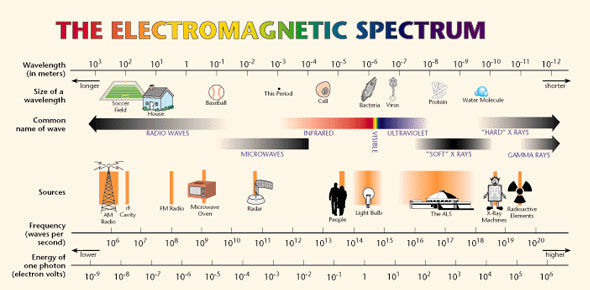# Electromagnetic Spectrum Quiz Questions

11 Questions | Total Attempts: 263SettingsA quiz over the NASA video "Video Tour Of The Electromagnetic Spectrum - 01 - Introduction"

• 1.
EMS stands for?
• A.

EletricoMovement Spectrum

• B.

ElectroMagnetic Spectrum

• C.

ElectroMagificent Spectrum

• D.

ElementaryMagetic Spectrum

• 2.
EM Waves travel at the constant speed of?
• A.

Light

• B.

Sound

• C.

Energy

• D.

Unknown

• 3.
The distance between crests is know as?
• A.

Crest Distance

• B.

Unknown Distance

• C.

Wavelength

• D.

Variable Distance

• 4.
Wavelengths are measured in?
• A.

Meters & Nanometers

• B.

Feet & Inches

• C.

Inches & Centimeters

• D.

Inches to CM Cal

• 5.
The amount of crests that pass a given point within one second is discribed as the?
• A.

Pass Point

• B.

Ultraviolet Pass Point

• C.

Crest Point Through

• D.

Frequency

• 6.
• A.

• B.

Wave Turn

• C.

Frequency

• D.

Point of Turn

• 7.
Microwaves carry?
• A.

Text messages

• B.

Atoms in food

• C.

Extra Waves in microwaves

• D.

Induction of waves in EO waves

• 8.
Color is seen because EM Waves interact with?
• A.

Other Waves in waves to make waves

• B.

Wide Bands of waves in water waves

• C.

Radar waves within waves to power waves

• D.

Molecules

• 9.
The visible light spectrum between 492NM and 577NM is interpreted as?
• A.

Green

• B.

Red

• C.

Blue mixed

• D.

NIR mixed

• 10.
EM radiation is emmitted, reflected and absorbed differently. A graph showing this interaction across the EM Spectrum is known as?
• A.

Signed of the Sign

• B.

Digital Wave

• C.

Spectral Signature

• D.

Wave Signature

• 11.
How long is this video?
• A.

4:30

• B.

5:10

• C.

5:03

• D.

3:43

Related TopicsBack to top